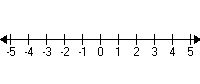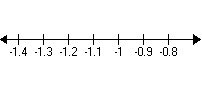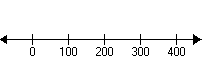# The Number Line

A number line is simply a representation of the ordering of the real numbers . Usually, we mark $0$ in the middle, the negative integers on the left, and the positive integers on the right:The arrow indicates that the line "keeps on going" in both directions.

When comparing numbers, the order in which they are placed on the number line will determine if one number is greater or less than another number.  In the above example, the numbers get smaller to the left and larger to the right.

Sometimes we may want to "zoom in" on a part of the number line, like this magnification of the part near $-1$ :or "zoom out" to show larger intervals like $10$ s or $100$ s: